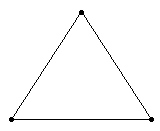Last updated: August 14, 2020

1. Varingon's theorem of moments states that if a number of coplaner forces acting on a particle are in equilibrium, then
2. In a screw jack, the effort required to lower the load is __________ the effort required to raise the same load.
3. The total energy possessed by a system of moving bodies
4. The linear acceleration (a) of a body rotating along a circular path of radius (r) with an angular acceleration of α rad / s2, is
5. A framed structure is imperfect, if the number of members are __________ (2j - 3).
6. The velocity of a particle moving with simple harmonic motion is __________ at the mean position.
7. The acceleration of a particle moving with simple harmonic motion is __________ at the mean position.
8. Energy may be defined as the capacity of doing work.
9. 1 joule is equal to
10. If the body falls freely under gravity, then the gravitational acceleration is taken as
11. The moment of inertia of a solid cylinder of mass m, radius r and length l about the longitudinal axis or polar axis is
12. Whenever a force acts on a body and the body undergoes a displacement, then
13. The periodic time (T) is given by(where ω = Angular velocity of particle in rad / s.)
14. A couple produces
15. For a self locking machine, the efficiency must be
16. The rate of change of displacement of a body is called
17. The terms 'leverage' and 'mechanical advantage' Of a compound lever have got the same meaning.
18. When a particle moves along a straight path, there will be centripetal acceleration as well as tangential acceleration.
19. A framed structure, as shown in the below figure, is a20. In a screw jack, the effort required to lift the load is given by(where W = Load lifted, α = Helix angle, and φ = Angle of friction.)
Engineering Mechanics MCQ 7
You got {{userScore}} out of {{maxScore}} correct
{{title}}
{{image}}
{{content}}